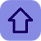# C Programming to Explain Type Casting

Posted by Tushar Bedekar

### Introduction:-

Type casting is a way to convert a variable from one data type to another data type. For example, if you want to store a long value into a simple integer then you can type cast long to int. You can convert values from one type to another explicitly using the cast operator as follows:

### Program me:-

#include< stdio.h>
#include< conio.h>
int main( )
{
int n,i,j,fact;
float sum=0;
clrscr();
for(i=1; i<=7; i++) { fact=1; for(j=i; j>=1; j--)                       // to find the factorial
fact=fact*j;

sum=sum+(float)i/fact;
}
printf("\nSum : %f",sum);
getch();
return 0;
}

#### 1 comment:

1.Hello , nice info aboutC Programming. thank you.